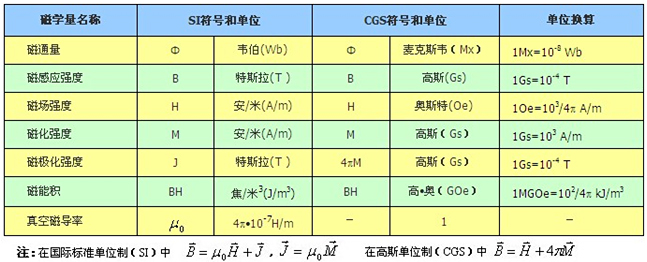hot line：0574-87939270
Classification
Magnet
 Magnetism of commonly used unit conversionPermanent magnetic material is plus after magnetization of magnetic field does not disappear, can provide a stable external space magnetic field. Ndfeb permanent magnets used measure has the following four:            Remanence (Br) units for the tesla (T) and gaussian (Gs) 1 Gs = 0.0001 T            Will be a magnet magnetization to technology by external magnetic field in a closed environment after saturated undo the external magnetic field, the magnetic induction intensity of magnet performance we call residual magnetism. It said magnet can provide maximum flux values. Visible from the demagnetization curve, it corresponds to the air gap is zero, so the actual magnetic induction intensity of magnetic circuit of the magnet are smaller than the residual magnetism. Ndfeb is now found in Br the highest practical permanent magnetic material.            Magnetic induction coercive force (Hcb) unit is/m (A/m) and oster (Oe) or 1 Oe 79.6 A/m material            In technology after saturation magnetization magnet magnetization, on the reverse of the magnetic induction intensity is zero the reverse magnetic field intensity values called magnetic induction coercive force (Hcb). But at the moment of magnet magnetization is not zero, but with the reverse magnetic field and magnet magnetization effects cancel each other out. (external magnetic induction intensity is zero) at this time if cancel the external magnetic field, magnets, still can have certain magnetic. Summary of coercive force is usually more than 11000 oe.            Intrinsic coercive force (Hcj) unit is/m (A/m) and oster (Oe) 1 Oe 79.6 A/m material            Make the magnet magnetization reverse magnetic field strength required for zero, we call it intrinsic coercive force. Intrinsic coercive force is measured magnet demagnetization resistance of a physical quantity, if the additional magnetic field is equal to the intrinsic coercive force magnets, magnet magnetic will basically eliminated. Summary of Hcj will decrease with the increase of temperature and so need to work in high temperature environment should choose high grade with Hcj.            Magnetic energy ((BH)  unit is focal/m 3 (J/m3) or high? Mr (GOe) 1 MGOe material 7. 96 k J/m3            Demagnetization curve on any bit of B and H is the product of BH we called magnetic energy product, and a maximum of B * H called the maximum magnetic energy product (BH) Max. Magnetic energy product is one of the important parameters of stored energy constant magnet size, (BH) Max shows that the greater the magnet is magnetic energy. Magnetic circuit design as far as possible to make the working point of magnets in near maximum magnetic energy product of B and H.            Isotropic magnet: any direction magnetic magnets can are the same.            Anisotropic magnets:magnetic can have different different directions; And there is a direction, the direction of orientation when the highest income magnetic magnets. Sintered ndfeb permanent magnet is anisotropic magnets.            Orientation direction:anisotropic magnets can obtain best referred to as the magnet in the direction of the magnetic orientation direction. Also known as "orientation axis", "easy magnetization axis".            The size of the magnetic field strength:refers to the space magnetic field somewhere, with H, said its unit is/m (A/m).            Magnetization:refers to the material inside the unit volume magnetic moment vector and, with M, said unit is/M (A/M).            Magnetic induction intensity:the definition of the magnetic induction intensity B is: B = 0 mu (H + M), in which H and M is the magnetization and magnetic field intensity, respectively and mu 0 is vacuum magnetic permeability. Also known as magnetic flux density, magnetic induction intensity of the magnetic flux per unit area. The unit is the tesla (T).            Flux:within a given area of the total magnetic induction intensity. When the magnetic induction intensity B evenly distributed in the surface of the magnet, the general formula for Φ flux Φ = B * A. Flux of SI unit is maxwell.            Relative permeability: medium permeability compared with the vacuum magnetic permeability ratio, namely the u r = u/u o. In the CGS system, mu o = 1. In addition, air relative permeability in be used actually tend to value is 1, and copper, aluminum and stainless steel materials of relative permeability is approximate to 1.            Permeability: the ratio of flux Φ and magnetomotive force F, similar to the circuit of the conductance. A physical quantity is reflect material permeability ability.            Permeability Pc:as the demagnetizing factor, again on the demagnetization curve, the ratio of the magnetic induction intensity and magnetic field intensity Hd Bd, namely Pc = Bd/Hd, permeability of conditions can be used to estimate the flux values. For isolated Pc only related to the size of the magnets, magnet demagnetization curve and Pc line intersection point is the working point of magnets, Pc magnet working point is higher, the greater the less easy to demagnetization. The general case for an isolated magnet orientation the bigger length relative to the Pc. So the Pc is an important physical quantities in the design of permanent magnet magnetic circuit.